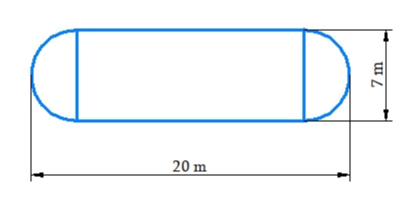# Ex.11.1 Q3 Mensuration Solutions - NCERT Maths Class 8

Go back to  'Ex.11.1'

## Question

The shape of a garden is rectangular in the middle and semicircular at the ends as shown in the diagram. Find the area and the perimeter of this garden [Length of rectangle is $$20 – (3.5 + 3.5)$$ meters].Video Solution
Mensuration
Ex 11.1 | Question 3

## Text Solution

What is Known?

Shape of a garden is rectangular in the middle and semicircular at the ends.

What is unknown?

Area and perimeter of the garden

Reasoning:

The garden is rectangular in the middle and semicircular at the ends. From the diagram, we can see that the area of the garden is the sum of the rectangular middle portion and two semicircles at the end.

Steps:

Length of the rectangular part

\begin{align} &= 20-\left( {3.5 + 3.5} \right){\rm{ meters}}\\ &= \left( {20 - 7} \right){\rm\;{ meters}}\\ &= 13{\rm{ meters}}\end{align}

Breadth of the rectangular part $$=7$$ meter

Area of the rectangular part

\begin{align} &= {\rm{length}} \times {\rm{breadth}}\\ &= 13\,{\rm\;{meter}} \times 7\,{\rm{meter}}\\ &= 91{\rm{ m}}{\rm{. sq}}{\rm{.}}\end{align}

Diameter of the semi circle $$= 7 \rm\,m$$

Radius of the semi circle =\begin{align}\frac{7}{2}\end{align}m $$= 3.5 \rm\,m$$

Area of the semi circle \begin{align} = \frac{1}{2} \times \pi \times {r^2}\end{align}

Area of two semi circles

\begin{align} &= 2 \times \frac{1}{2} \times \frac{{22}}{7} \times {(3.5)^2}\\&= \frac{{22}}{7} \times 12.25\\ &= 38.5\,\,{{\rm{m}}^2}\end{align}

Total area of the garden = (Area of rectangle) + (Area of two semicircle regions)

\begin{align}& = 91{{\rm{m}}^2} + 38.5{{\rm{m}}^2}\\ &= 129.5{{\rm{m}}^2}\end{align}

Perimeter of the garden = length of rectangle + perimeter of two semicircle + length of rectangle

\begin{align}&= \,13 + 2\left( {\pi \times 3.5} \right) + 13\\&= \,26 + 7\pi \\&= \,26 + \not\!{7}\times \frac{{22}}{{\not\!{7}}}{\rm{m}}\\&= \,26 + 24{\rm{m}}\\&= \,48{\rm\;{m}}\end{align}

Learn from the best math teachers and top your exams

• Live one on one classroom and doubt clearing
• Practice worksheets in and after class for conceptual clarity
• Personalized curriculum to keep up with school